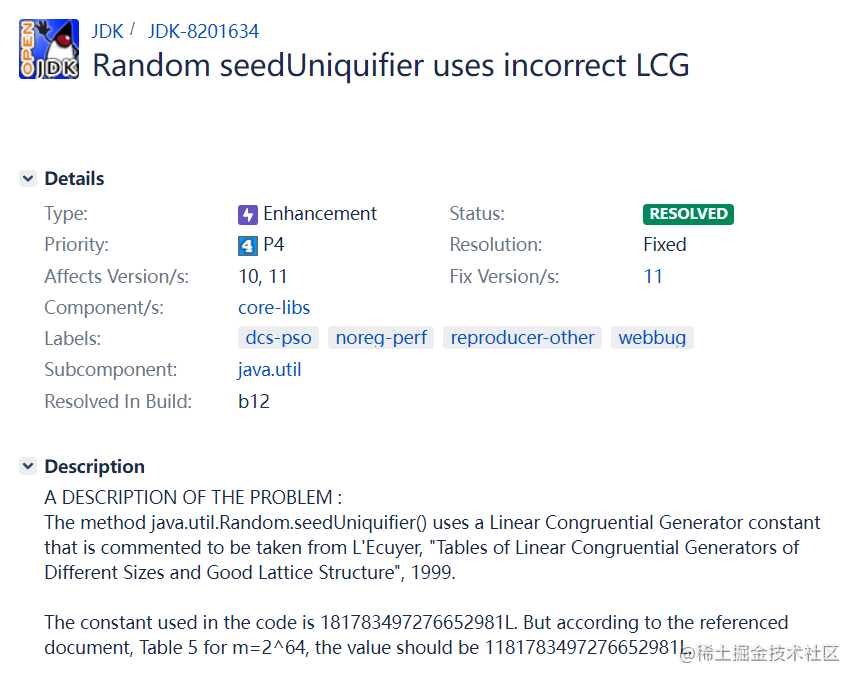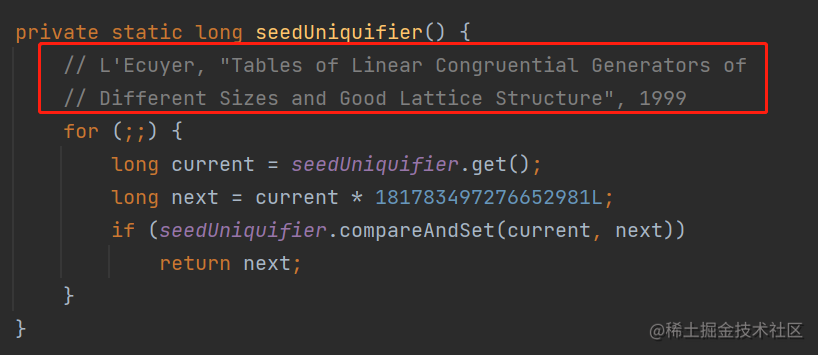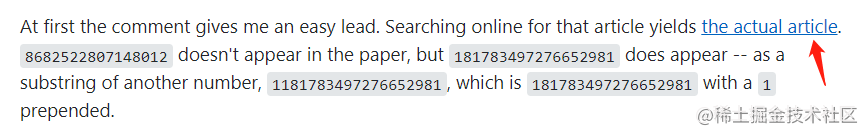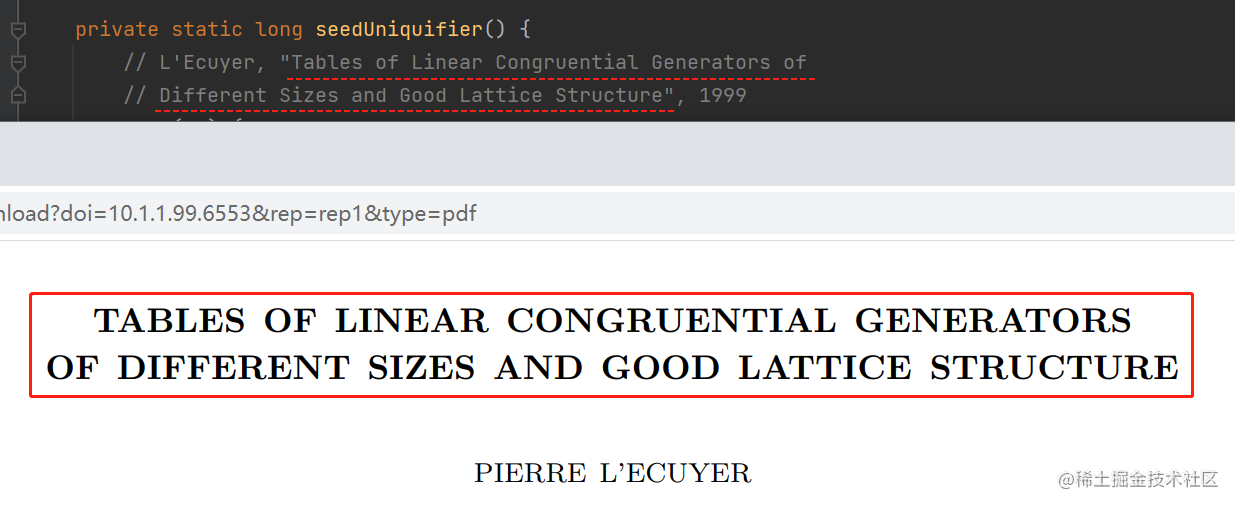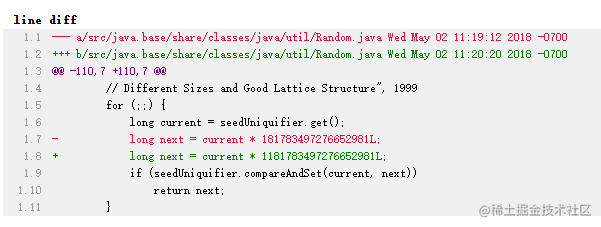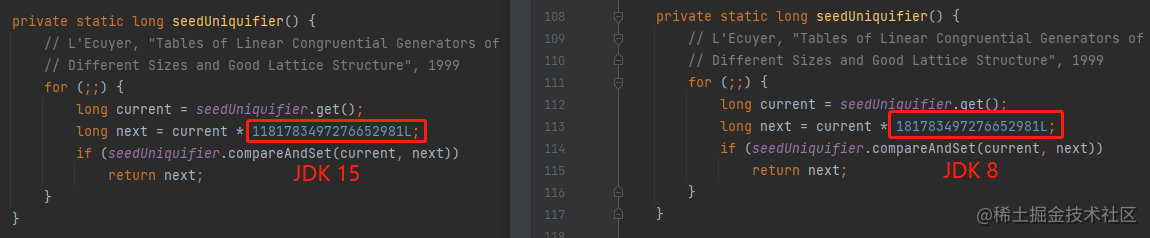# 初看一脸懵逼，看懂直接跪下！stackoverflow.com/questions/1…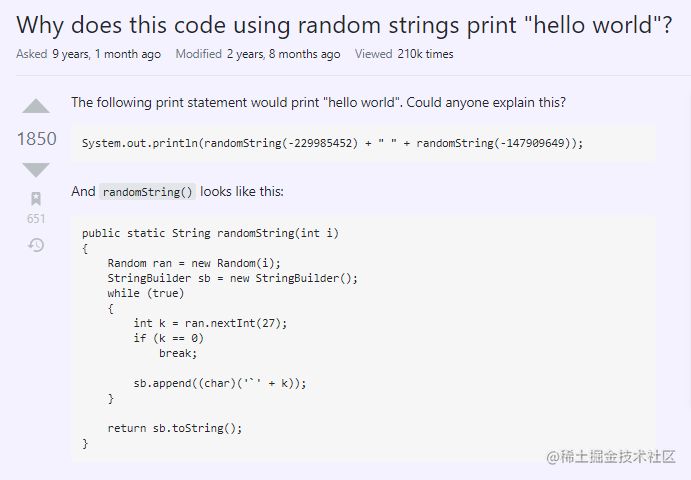``````public class MainTest {

public static void main(String[] args) {
System.out.println(randomString(-229985452) + " " + randomString(-147909649));
}

public static String randomString(int i) {
Random ran = new Random(i);
StringBuilder sb = new StringBuilder();
while (true) {
int k = ran.nextInt(27);
if (k == 0)
break;

sb.append((char) ('`' + k));
}
return sb.toString();
}
}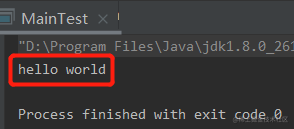## 高赞回答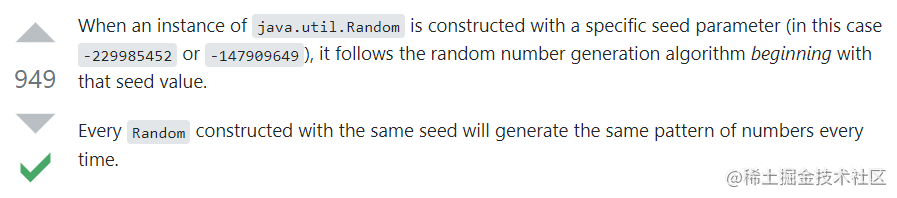``````public static void main(String[] args) {

randomString(-229985452);
System.out.println("------------");
randomString(-229985452);

}

private static void randomString(int i) {
Random ran = new Random(i);
System.out.println(ran.nextInt());
System.out.println(ran.nextInt());
System.out.println(ran.nextInt());
System.out.println(ran.nextInt());
System.out.println(ran.nextInt());

}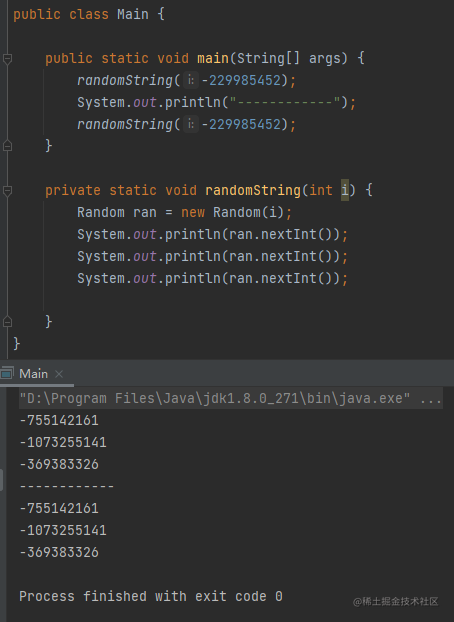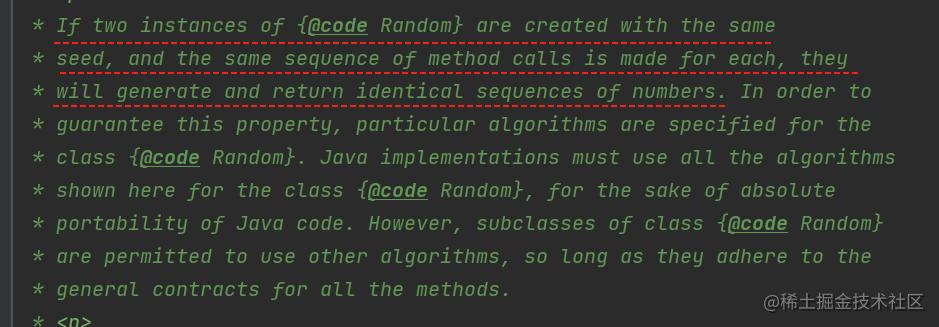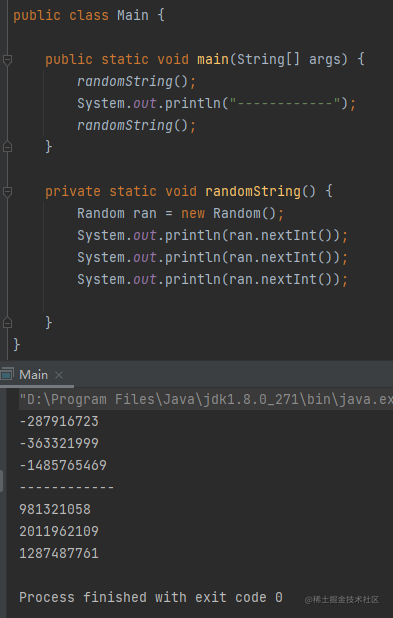## 再看看问题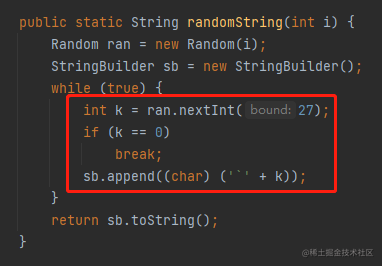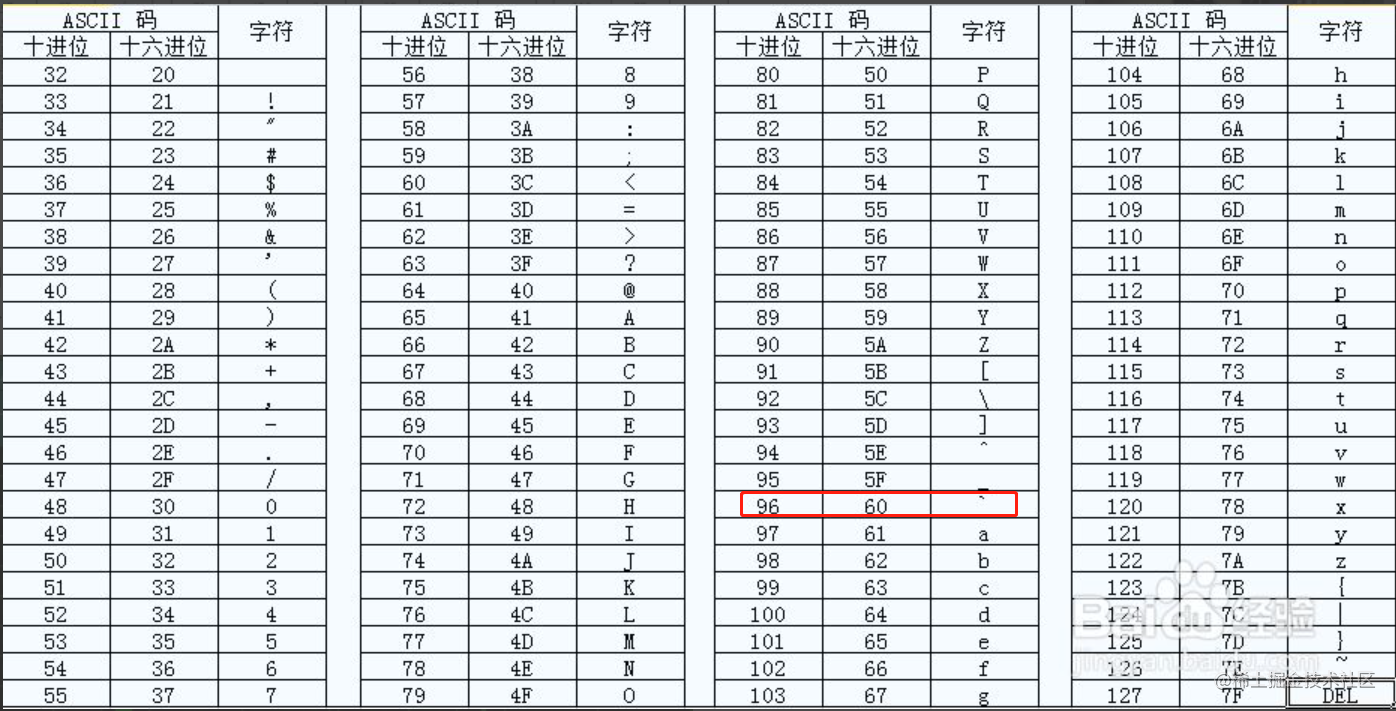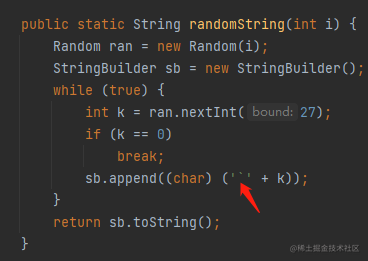'`' + k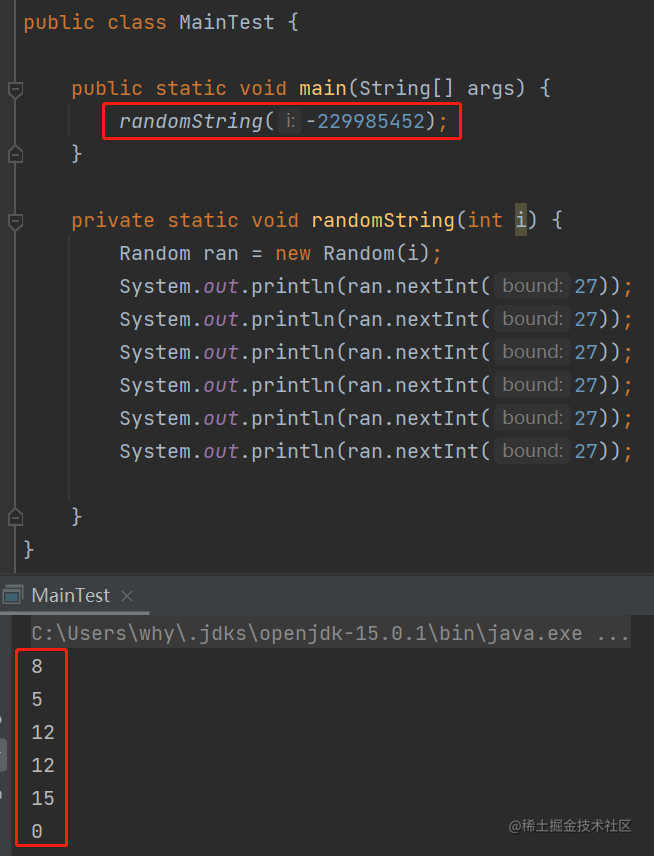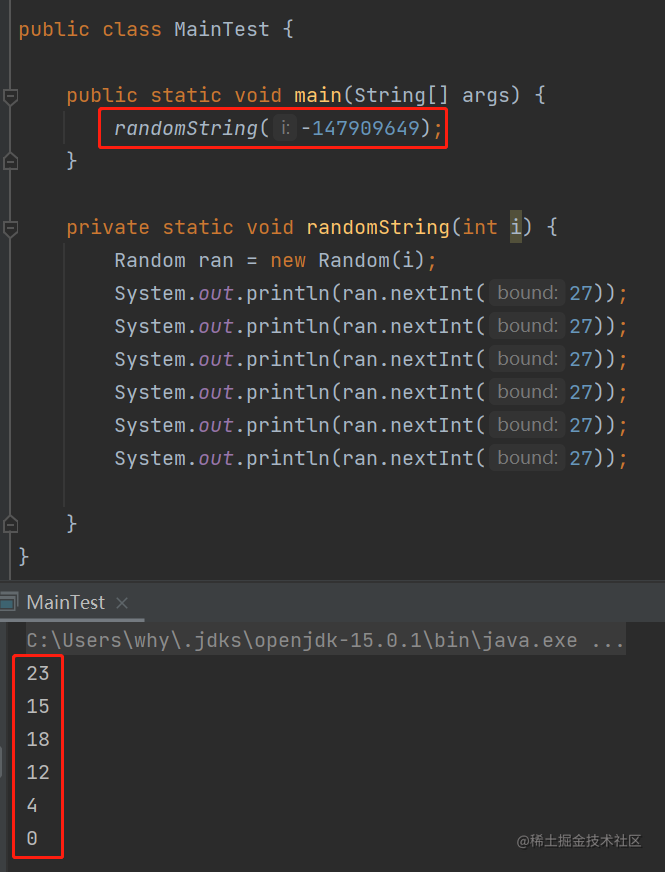8 + 96 = 104 --> h
5 + 96 = 101 --> e
12 + 96 = 108 --> l
12 + 96 = 108 --> l
15 + 96 = 111 --> o

23 + 96 = 119 --> w
15 + 96 = 111 --> o
18 + 96 = 114 --> r
12 + 96 = 108 --> l
4 + 96 = 100 --> d\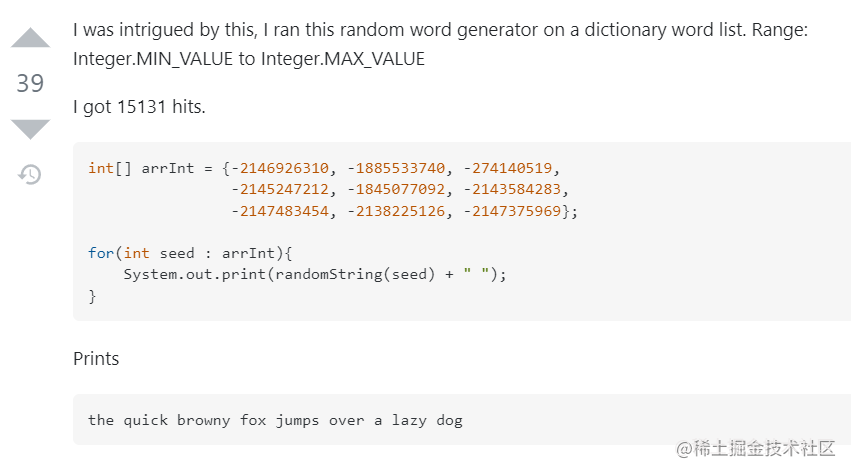``````public static long generateSeed(String goal, long start, long finish) {
char[] input = goal.toCharArray();
char[] pool = new char[input.length];
label:
for (long seed = start; seed < finish; seed++) {
Random random = new Random(seed);

for (int i = 0; i < input.length; i++)
pool[i] = (char) (random.nextInt(27) + '`');

if (random.nextInt(27) == 0) {
for (int i = 0; i < input.length; i++) {
if (input[i] != pool[i])
continue label;
}
return seed;
}
}
throw new NoSuchElementException("Sorry :/");
}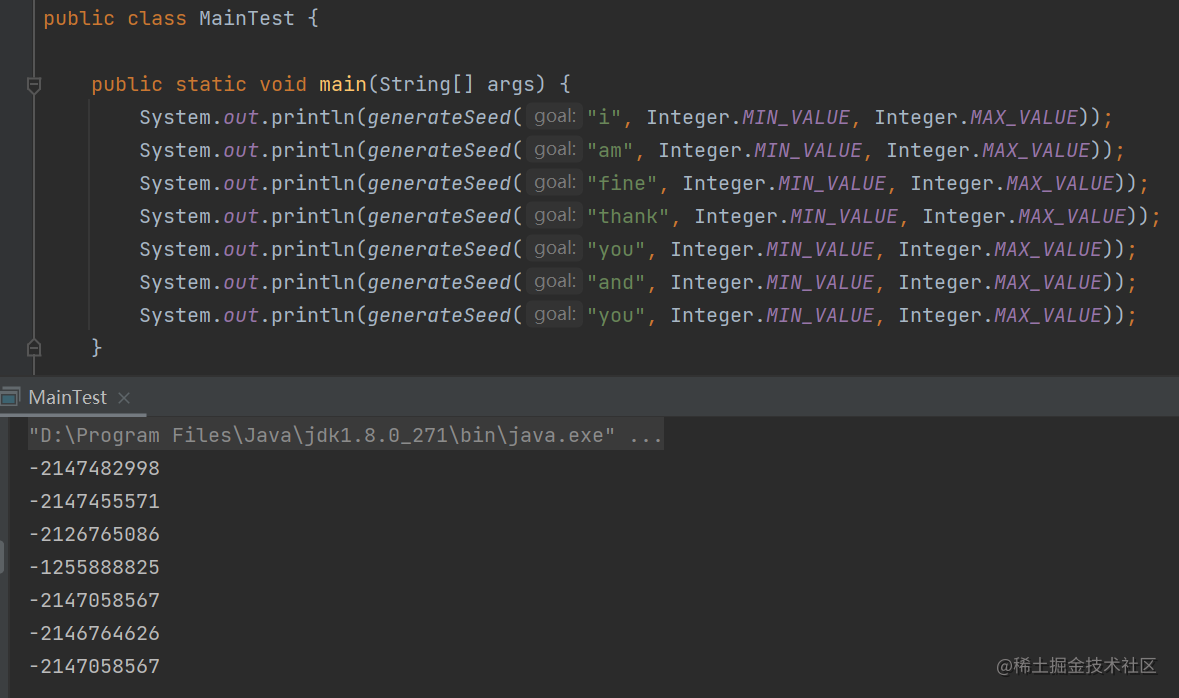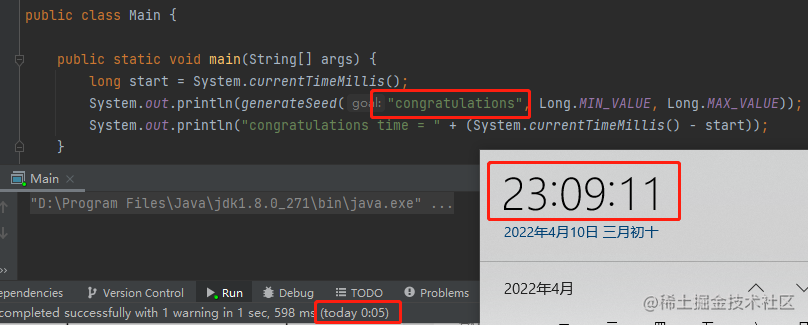但是理论上来讲只要有足够长的时间，这个 seed 一定会被找到。## 源码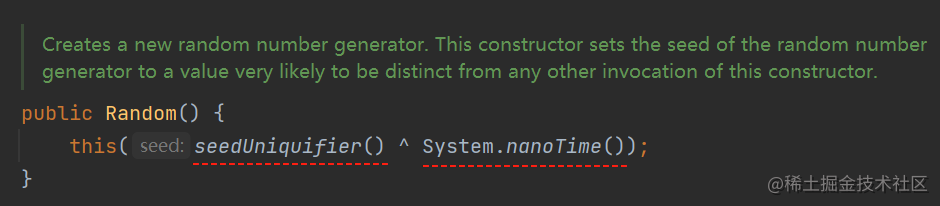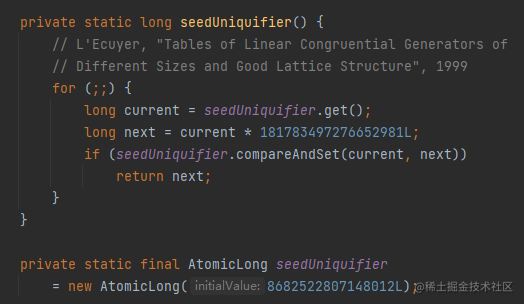181783497276652981L
8682522807148012L

stackoverflow.com/questions/1…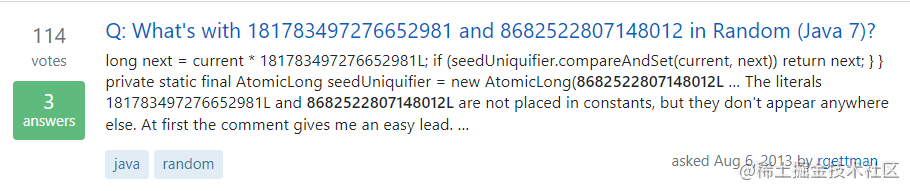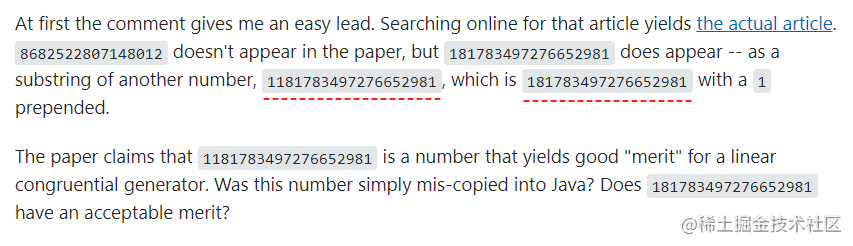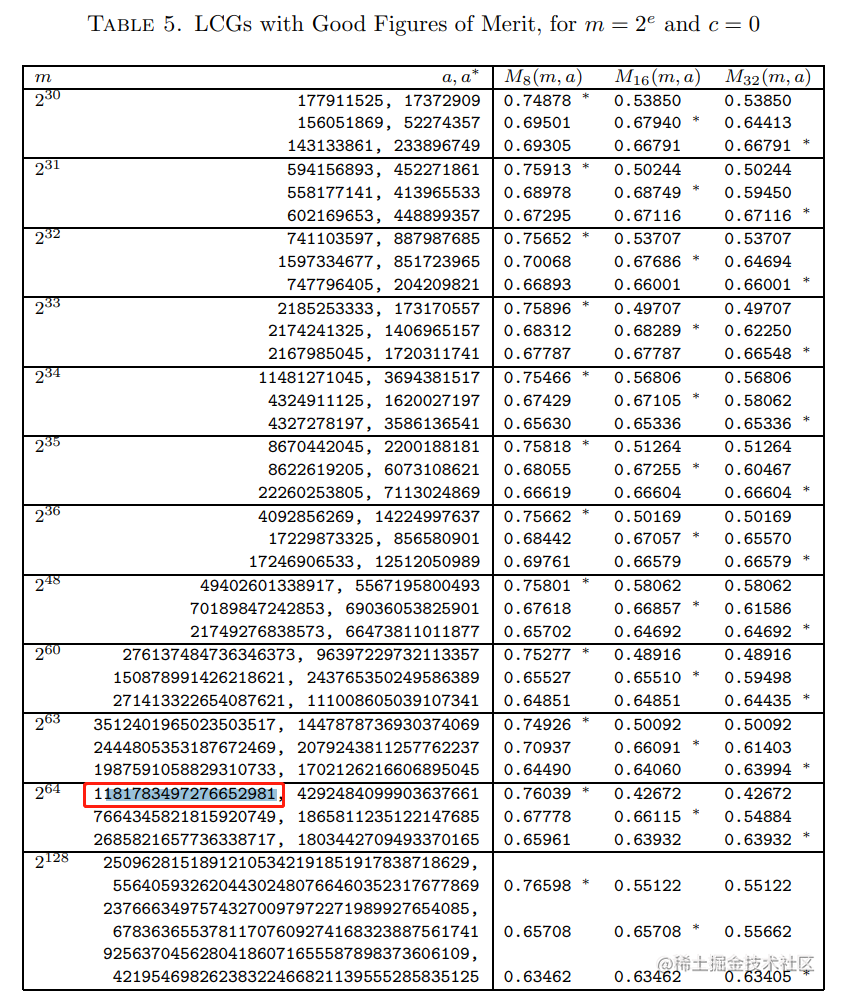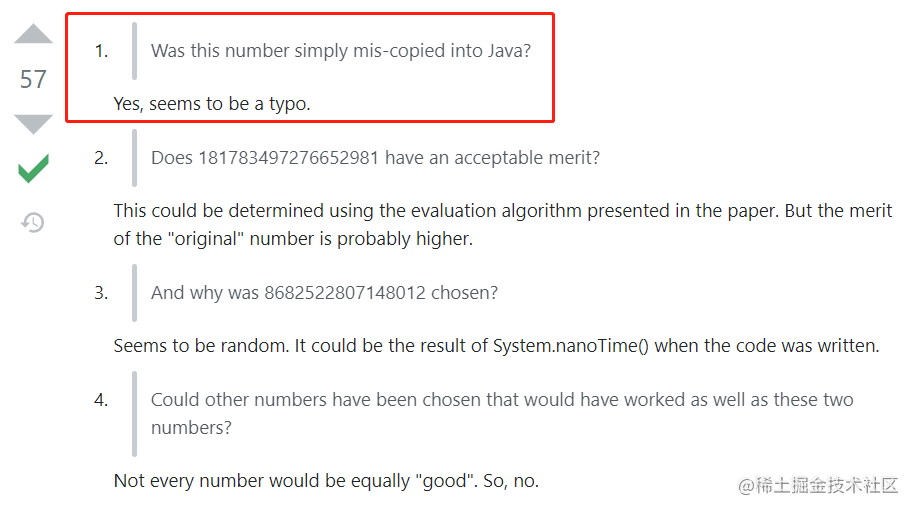“看起来确实像是拷错了。”bugs.openjdk.java.net/browse/JDK-…